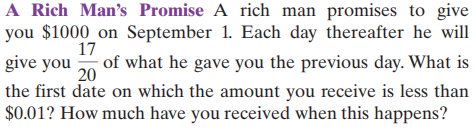### ¿Todavía tienes preguntas de matemáticas?

Pregunte a nuestros tutores expertos
Algebra
PreguntaA Rich Man's Promise

A rich man promises to give you $$\ 1000$$ on September $$1$$ . Each day thereafter he will give you $$\frac { 17 } { 20 }$$ of what he gave you the previous day. What is the first date on which the amount you receive is less than $$\ 0.01$$ ? How much have you received when this happens?

this forms a GP with common ratio = $$\frac{17}{20}$$

(a) $$\frac{1}{100}= 1000\times (\frac{17}{20})^{n- 1}$$

$$n\approx 71.84052$$

n = 72

(b) 6666.67

Solución
View full explanation on CameraMath App.